Shipra Saxena — Published On September 16, 2021 and Last Modified On September 10th, 2022

### Objective

• Understand what is Cross-sell using Vehicle insurance data.
• Learn how to build a model for cross-sell prediction.

### Introduction

If you are a Machine learning enthusiast or a data science beginner, it’s important to have a guided journey and also exposure to a good set of projects.In this article, We will walk through a beginner project in machine learning on cross-sell prediction. It will show you a basic approach to solve a predictive problem.

This project is inspired from my learnings from a very comprehensive free course that Analytics Vidhya recently launched. You can find the link below-

So let’s dive into the project.

## What is Cross-Sell Prediction?

It is important to understand the problem domain and key terms used in the definition of a problem before beginning a project. In the financial services industry, cross-selling is a popular term.

Cross-selling involves selling complementary products to existing customers. It is one of the highly effective techniques in the marketing industry.

To understand better, suppose you are a bank representative and you try to sell a mutual fund or insurance policy to your existing customer. The main objective behind this method is to increase sales revenue and profit from the already acquired customer base of a company.

Cross-selling is perhaps one of the easiest ways to grow the business as they have already established a relationship with the client. Further, it is more profitable as the cost of acquiring a new customer is comparatively higher.

## Problem Statement for Cross-Sell Prediction Problem

In this project, our client is an insurance company XYZ limited that has provided Health Insurance to its customers. Now, They want to build a model to predict whether the policyholders from the past year will also be interested in Vehicle Insurance provided by the company.

Developing a model to estimate whether a customer will be interested in a vehicle insurance policy is extremely helpful for the company. This would enable the organization to plan its communication strategy so that it can reach out to these customers and optimize its business model.

The problem statement and the dataset can be accessed from the Analytics Vidhya data hack platform.

The problem definition specifies that in order to predict, whether the customer would be interested in Vehicle insurance, we have information about demographics (gender, age, region code type), Vehicles (Vehicle Age, Damage), Policy (Premium, sourcing channel) etc.

## Hypothesis Generation for Cross-Sell Prediction

Once you have understood the problem statement and gathered the required domain knowledge. The next step comes, the hypothesis generation. This will directly spring from the problem statement. Whatever set of analysis we can think of at this stage we should write it down.

The structured thinking approach will help us here. Let me state some hypotheses from our problem statement.

1. Male customers are more tend to buy vehicle insurance than females.
2. The middle-aged customers would be more interested in the insurance offer.
3. Customers having a driving license are more prone to convert.
4. Those with new vehicles would be more interested in getting insurance.
5. The customers who already have vehicle insurance won’t be interested in getting another.
6. If the Customer got his/her vehicle damaged in the past, they would be more interested in buying insurance.

The above mentioned are just a few examples of hypothesis generation. You are free to add as many you want. Once you have the hypothesis ready at your end, it’s time to look into data and validate the statements.

## Implementation of Cross Sell Prediction in Python

In this section, we will have the implementation of our project. We have downloaded the dataset from the data hack platform in the form of a CSV file.  Let’s read the data and see what is there for us.

#### Import Libraries

```import pandas as pd

import numpy as np

import matplotlib.pyplot as plt

import seaborn as sns

!pip install imblearn

from sklearn.metrics import accuracy_score, f1_score,auc

from sklearn.linear_model import LogisticRegression

from sklearn.tree import DecisionTreeClassifier

from sklearn.model_selection import GridSearchCV

from sklearn.ensemble import RandomForestClassifier```

The first step is to look at the top 5 rows in the dataframe. This will give us an initial picture of the data.

```df= pd.read_csv('/content/train_data.csv')
df.shape

Hit Run to see the output

`df.info()`

Here, we will see the basic details of the features in the given dataset. Like the columns, non-null values in each column, and the respective data type.

In this dataset, we have 12 columns of different data types like int64, float64, and object.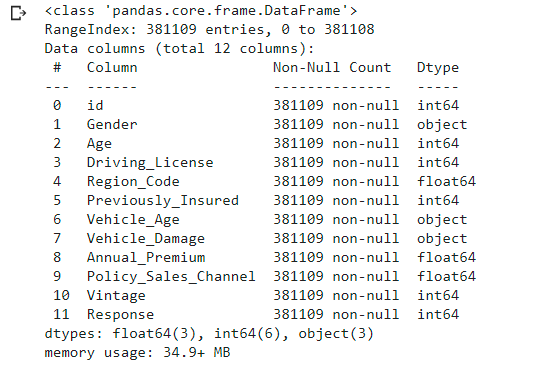Now, we will look for any of the missing values in the given dataset.

```df.isna()

```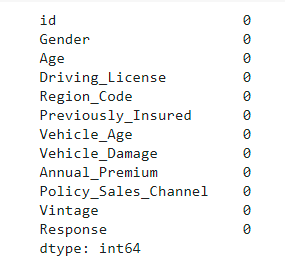We don’t have any missing values in this data. Hence we can move forward to the Exploratory data analysis step.

#### Exploratory Data Analysis

Before jumping into modelling and creating a machine learning-based solution for the given problem, it is important to understand the basic traits of the data.

For example, what is the distribution of numerical features? Also, EDA plays a part in validating our hypothesis.

fig, axes = plt.subplots(2, 2, figsize=(10, 10))

```sns.countplot(ax=axes[0,0],x='Gender',hue='Response',data=df,palette="mako")
sns.countplot(ax=axes[1,0],x='Previously_Insured',hue='Response',data=df,palette="mako")
sns.countplot(ax=axes[1,1],x='Vehicle_Age',hue='Response',data=df,palette="mako")```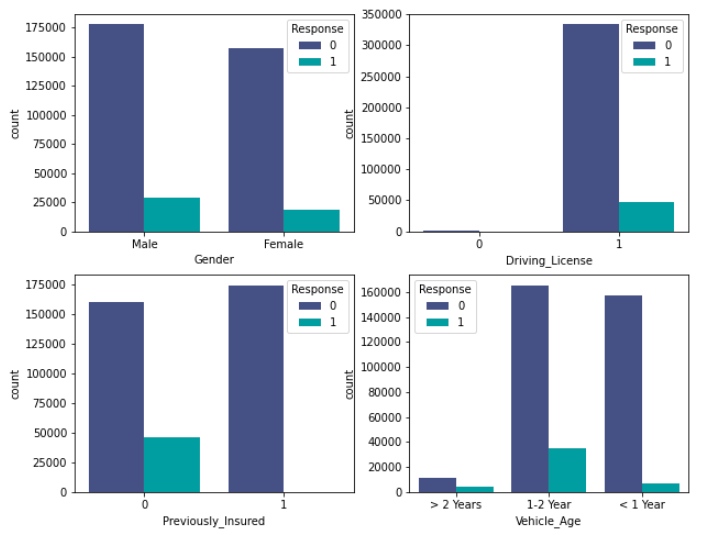From the above visualizations, we can make the following inferences.

The male customers own slightly more vehicles and they are more tend to buy insurance in comparison to their female counterparts.

Similarly, the customers who have driving licences will opt for insurance instead of those who don’t have it.

The third visualization depicts that the customers want to have only an insurance policy. It means those who already have insurance won’t convert.

In the last chart, the customers with vehicle age lesser than the 2 years are more tend to buy insurance.

```sns.countplot(x='Vehicle_Damage',hue='Response',data=df,palette="mako")

```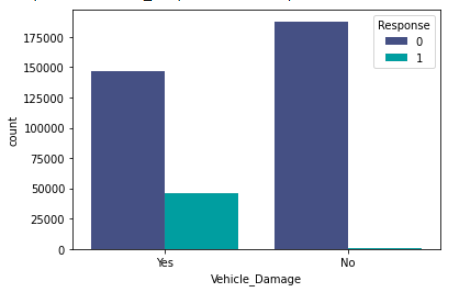From the above plot, we can infer that if the vehicle has been damaged previously then the customer will be more interested in buying the insurance as they know the cost.

It is also important to look at the target column, as it will tell us whether the problem is a balanced problem or an imbalanced problem. This will define our approach further.

The given problem is an imbalance problem as the Response variable with the value 1 is significantly lower than the value zero.

```Response = df.loc[:,"Response"].value_counts().rename('Count')
plt.xlabel("Response")
plt.ylabel('Count')
sns.barplot(Response.index , Response.values,palette="mako")```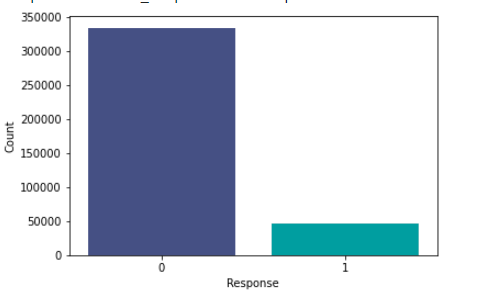Here, we have the distribution of the age. Most of the customers fall in the 20 to 50 age range. Similarly, we can see the distribution of annual premium

`sns.displot(df['Age'])`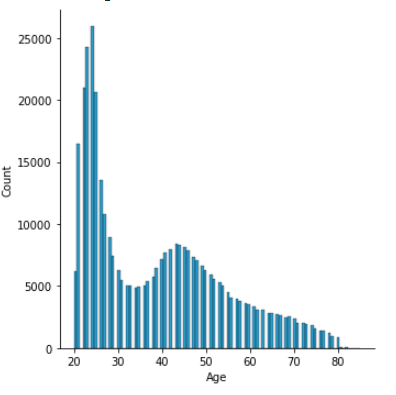```sns.distplot(df['Annual_Premium'])
```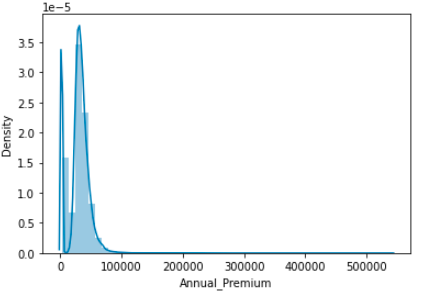#### Data preprocessing

The next step in the project is to prepare the data for the modelling. The following preprocessing techniques are being used here

1. Convert the categorical features into dummies or doing categorical encoding.
2. Binning the numerical features.
3. dropping the unnecessary columns like ids.

Here we a have user-defined function. We just need to pass the raw dataframe and we will get the preprocessed one.

```def data_prep(df):

df= df.drop(columns=['id','Policy_Sales_Channel','Vintage'])

df=pd.get_dummies(df,columns=['Gender'] ,prefix='Gender')

df=pd.get_dummies(df,columns=['Vehicle_Damage'] ,prefix='Damage')

df=pd.get_dummies(df,columns=['Previously_Insured'] ,prefix='prev_insured')

df["Age"] = pd.cut(df['Age'], bins=[0, 29, 35, 50, 100])

df['Age']= df['Age'].cat.codes

df['Vehicle_Age'] =df['Vehicle_Age'].map({'< 1 Year': 0, '1-2 Year': 1, '> 2 Years': 2})

df.drop(columns=['Region_Code'],inplace= True)

return df
df1=data_prep(df)```
`df1.head()`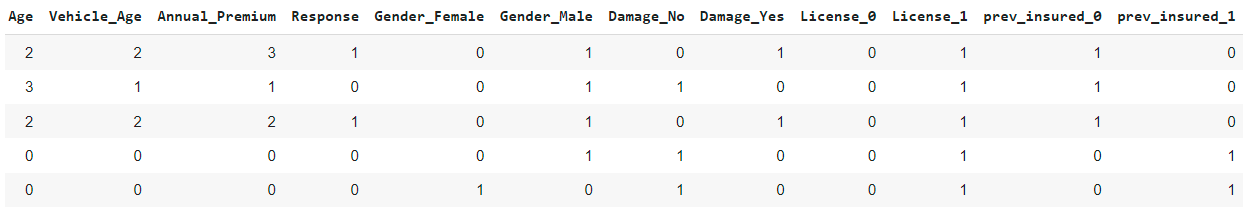#### Select Feature

In the following code, we will select those features only we want to use in our model training.

```Features= ['Age','Vehicle_Age','Annual_Premium',"Gender_Female","Gender_Male","Damage_No","Damage_Yes",

#### Train-Test split

In the next step, we will split the whole data in our hands into train data and test data.

The train data, as the name suggests will be used for training our machine learning model. On the other hand test, data will be used to make predictions and test the trained model.

Here, I have kept 30% of the total data for testing and the remaining 70% will be used for model training.

```from sklearn.model_selection import train_test_split
X_train, X_test, Y_train, Y_test = train_test_split(df1[Features],df1['Response'],
test_size = 0.3, random_state = 101)
X_train.shape,X_test.shape```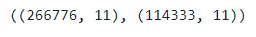#### Handle Imbalance Data Problem

As from the distribution of target variables in the EDA section, we know it is an imbalance problem.  The imbalance datasets could have their own challenge.

For example, a disease prediction model may have an accuracy of 99% but it is of no use if it can not classify a patient successfully.

So to handle such a problem, we can resample the data. In the following code, we will be using undersampling.

Undersampling is the method where we will be reducing the occurrence of the majority class up to a given point.

```from imblearn.under_sampling import RandomUnderSampler

RUS = RandomUnderSampler(sampling_strategy=.5,random_state=3,)

X_train,Y_train  = RUS.fit_resample(df1[Features],df1['Response'])```

#### Cross-Sell Prediction – Model training and prediction

Now, it is time to train a model and make predictions.  Here,  I have written a user-defined function for measuring the performance of the models.

For performance measurement, we will be using the accuracy score and F1 score. It is important to note here that for imbalanced classification problems, the F1 score is a more significant metric.

def performance_met(model,X_train,Y_train,X_test,Y_test):

```    acc_train=accuracy_score(Y_train, model.predict(X_train))

f1_train=f1_score(Y_train, model.predict(X_train))

acc_test=accuracy_score(Y_test, model.predict(X_test))

f1_test=f1_score(Y_test, model.predict(X_test))

print("train score: accuracy:{} f1:{}".format(acc_train,f1_train))

print("test score: accuracy:{} f1:{}".format(acc_test,f1_test))```

In this section, first, we will train three models

• Logistic Regression
• Decision Tree
• Random Forest

#### Logistic Regression

```model = LogisticRegression()
model.fit(X_train,Y_train)
performance_met(model,X_train,Y_train,X_test,Y_test)

```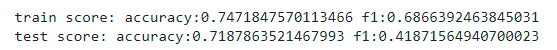#### Decision Tree

```model_DT=DecisionTreeClassifier(random_state=1)
model_DT.fit(X_train,Y_train)
performance_met(model_DT,X_train,Y_train,X_test,Y_test)```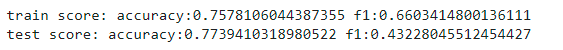#### Random forest

```Forest= RandomForestClassifier(random_state=1)
Forest.fit(X_train,Y_train)
performance_met(Forest,X_train,Y_train,X_test,Y_test)```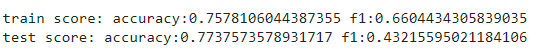In all models, the performance of the logistic regression model is significantly low, whereas the decision tree and random forest models are showing approximately the same performance.

#### Hyperparameter tuning

For this project, the last step is to do some hyperparameter tuning. It is a process to find the best performing hyper-parameters.

Here, we will be using a GridSearch algorithm for finding the best parameters of a random forest classifier.

```rf= RandomForestClassifier(random_state=1)

parameters = {

'bootstrap': [True],

'max_depth': [20, 25],

'min_samples_leaf': [3, 4],

'min_samples_split': [100,300],

}

grid_search_1 = GridSearchCV(rf, parameters, cv=3, verbose=2, n_jobs=-1)

grid_search_1.fit(X_train, Y_train)

performance_met(grid_search_1,X_train,Y_train,X_test,Y_test)```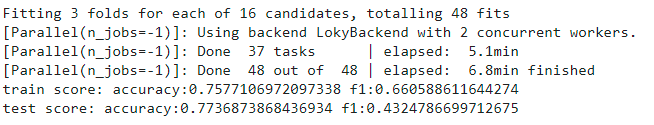We can see that after using some basic hyperparameter tuning, the f1 score has slightly improved. You can take this further and try to improve the performance of the model.

## Conclusion

This article explained cross-sell prediction comprehensively. Cross-sell prediction is a very common machine learning problem that is relevant in the industry.

This is a basic machine learning project that I did in the initial days of my data science journey. If you are a newbie in machine learning, it’s essential for you to have hands-on experience with some projects.

If you want to learn machine learning from scratch here we have a free course for you-